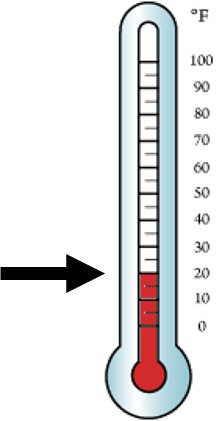1
visibility

What is the temperature shown on the given thermometer?• \$latex 20^\circ F\$

• \$latex 30^\circ F\$

• \$latex 15^\circ F\$

The correct answer is  20°F.

Solution:

First, we find the point where the thermometer changes from red to white.Then, we look at the number to the right.

The temperature is  20°F  as desired.